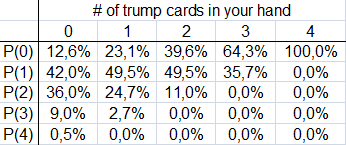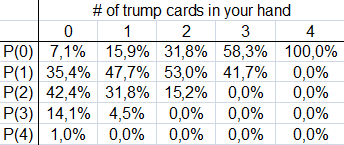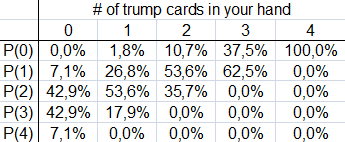## 2010-10-07

### Probabilities

In this post, I am going to provide the most important probabilities in Schnapsen. Please note that the following tables are correct only as long as players receive additional cards from the deck after each trick. So if the deck is closed, these probabilities do not apply.

The following table displays the probabilities at the start of the game:This table tells you the probabilities of your opponent holding x trumps cards (P(x)) given that you have a certain number of trump cards in your hand (this number is listed in the second row).

Example #1. If you have 2 trump cards in your starting hand, your opponent is 39.6% to have no trump cards at all.

While the primary purpose of these tables is to show the probabilities associated with trump cards, you can derive many other probabilities.

Example #2. A certain strategy option you have only works if your opponent does not have one specific card. How likely is this scenario? Basically, this situation is the same as the following one: You have 3 trump cards and your opponent has the sole remaining trump card. Therefore, the probability of your opponent having the card is 35.7%. This strategy option will work 64.3% of the time.

Example #3. A certain strategy option only works if your opponent does not have 2 specific cards at the same time (if he only has one of them, you will still succeed). Mathematically, this situation is equivalent to you having 2 trump cards and your opponent not having the remaining 2 trump cards. Therefore, this strategy option is going to work 89.0% of the time.

Example #4. You would prefer your opponent not to have 3 specific cards. This is equivalent to you having one trump card and your opponent not having any other trump card. So, the associated probability is 23.1%.

Example #5. This example might not be relevant in an actual playing situation, but it helps you understand how to use the probability tables: The 2 cards of interest are the Ad and the Tc. What is the probability of your opponent holding the Tc while not having the Ad? Your opponent is going to have the Tc 35.7% of the time. Furthermore, he will have both the Tc and the Ad 11.0% of the time. Consequently, your opponent is 24.7% to hold the Tc without having the Ad.

During a game, these probabilities change. After the first trick, the probability table looks like this, given that all trump cards are still live:Now, let's say that one trump card has left the game. Then, the table would look like this:As you can see, the probabilities are the same, but have moved one column to the left. So, it is rather easy to determine the tables if trump cards are out of the game: You move the figures to the left by as many columns as trump cards are out of the game.

After the second trick, the table looks like this:Please note that your opponent is exactly 50% to have any one specific card at this point.

The probabilities after the third trick are the following ones:Finally, after the fourth trick, the table looks like this:While it might not be necessary to exactly know all of these figures, you should be able to roughly estimate how (un)likely certain scenarios are. This will help you estimate the winning percentages of strategy decisions more accurately.